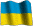Journal
of Numerical
and Applied
Mathematics

Main Page

Editorial Board

Instruction for Authors

Publication Ethics

Abstracts# Journal of Numerical and Applied Mathematics

### (Series "Numerical Mathematics")

Main Editor of Series

academician of NASU, prof. V.L. Makarov
Institute of Mathematics, NASU
makarov@imath.kiev.ua
(numerical functional analysis, numerical methods
for evolution problems and eigenvalues problems,
theory of difference schemes)

Associate Editors of Series:

prof. M.M. Voytovich
Pidstrygach Institute for Applied Problems of Mechanics and Mathematics, NASU
voi@iapmm.lviv.ua
(nonlinear integral equation, direct and inverse scattering problems,
numerical methods of spectral problems)

prof. I.P. Gavrilyuk
ipg@ba-eisenach.de
(numerical functional analysis, numerical methods for ODE,
numerical methods for PDE, fast algorithms,
mathematical modelling in hydrodynamics)

prof. M.V. Kutniv
National University "Lviv Polytechnics"
kutniv@yahoo.com
( numerical methods for ODE, exact compact difference schemes
and compact difference schemes with high order
for boundary value problems for ODE)

prof. P.P. Matus
Department of Mathematics, the John Paul II Catholic University of Lublin, Poland,
Institute of Mathematics, National Academy of Science of Belarus
matus@im.bas-net.by
http://www.cmam.info/matus/
(nonlinear stability of difference schemes for equations of gasdynamics,
exact difference schemes for time-dependent problem,
mathematical modelling of technological processes at an oil refining factory)

prof. S.G. Solodkyy
Institute of Mathematics, NASU
solodky@imath.kiev.ua
( ill posed problems and informational complexity, regularization methods,
strategy of choice of regularization parameters, optimal discretization,
stability and convergence of numerical methods,
error estimates of reconstruction of solutions)

prof. R.S. Chapko
Ivan Franko National University of Lviv
chapko@is.lviv.ua
( numerical solution of integral equations, integral equation method,
numerical solution of inverse problems)

prof. O.M. Khimich
Glushkov Institute of Cybernetics, NASU
dept150@insyg.kiev.ua
khimich_ic@mail.ru
(mathematical models with approximate input data)

prof. V.V. Khlobystov
Institute of Mathematics, NASU
khl@i.com.ua
(approximation of functions and operators)

prof. G.A. Shynkarenko
Ivan Franko National University of Lviv
gashynk@lnu.edu.ua
h.shynkarenko@po.opole.pl
(construction, analysis and application of the finite element method)